# 无监督学习：寻求数据的表示形式

## 聚类：对观察数据进行分组

### K-均值(K-means)聚类``````>>> from sklearn import cluster, datasets

>>> k_means = cluster.KMeans(n_clusters=3)
>>> k_means.fit(X_iris)
KMeans(n_clusters=3)
>>> print(k_means.labels_[::10])
[1 1 1 1 1 0 0 0 0 0 2 2 2 2 2]
>>> print(y_iris[::10])
[0 0 0 0 0 1 1 1 1 1 2 2 2 2 2]
``````

k_means 算法不能保证运行结果跟真实情况相同。首先，很难选择聚类的个数。其次，由于该算法对初始化很敏感，并且可能会有局部最优解，尽管scikit-learn使用了几种技巧来减少以上问题的发生。8个聚类``````>>> import scipy as sp
>>> try:
...    face = sp.face(gray=True)
... except AttributeError:
...    from scipy import misc
...    face = misc.face(gray=True)
>>> X = face.reshape((-1, 1)) # 我们需要一个形状为(n_sample, n_feature)的数组
>>> k_means = cluster.KMeans(n_clusters=5, n_init=1)
>>> k_means.fit(X)
KMeans(n_clusters=5, n_init=1)
>>> values = k_means.cluster_centers_.squeeze()
>>> labels = k_means.labels_
>>> face_compressed = np.choose(labels, values)
>>> face_compressed.shape = face.shape
``````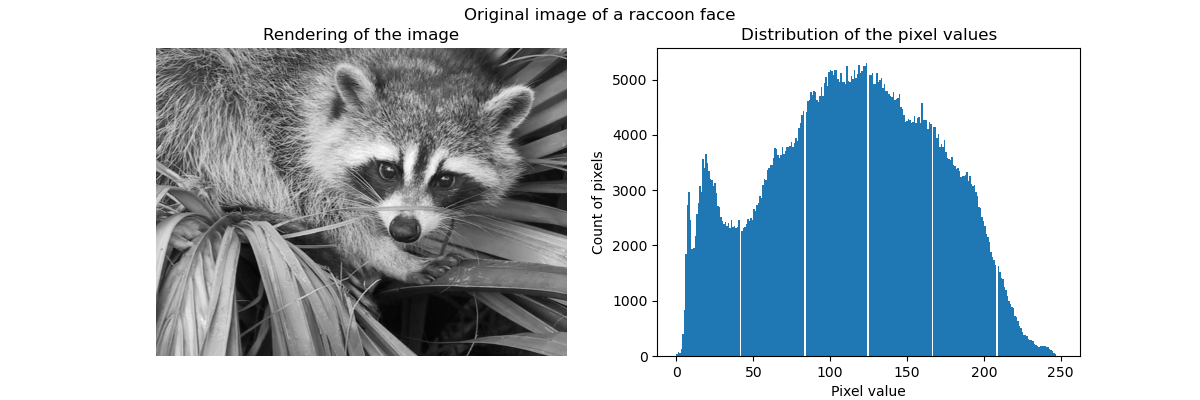K均值量化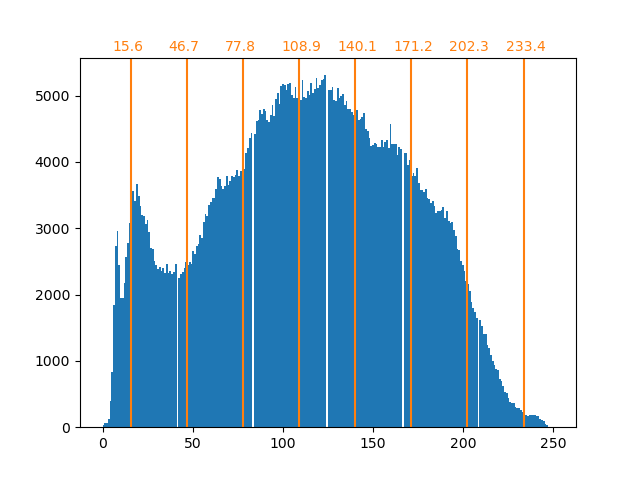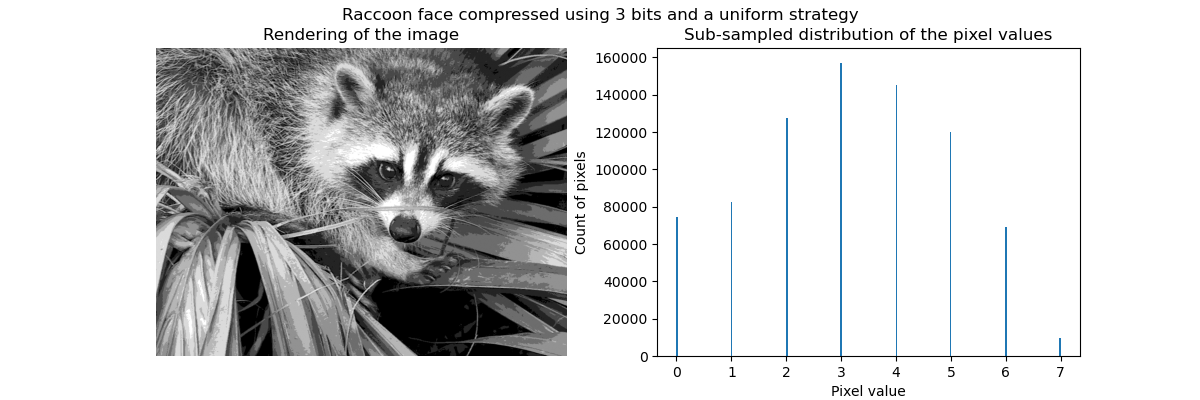### 分层聚合聚类: Ward

• 聚合(Agglomerative) -自下而上的方法：每个观察数据都从单独一个聚类开始，并且以最小化距离标准的方式进行迭代地合并。当仅由少量观察数据组成时，此方法会引起人们的注意。当聚类个数很大时，它的计算效率比k均值高效地多。
• 分裂(Divisive) - 自上而下的方法：所有的观察数据都开始于同一个聚类，当一个聚类向下一层移动时，它会被迭代地拆分。当对大量的数据进行估计时，此方法不仅速度慢（由于所有观察都是从同一个聚类开始，然后递归拆分），而且从统计学的角度来讲也是不合适的。

#### 连接约束聚类(Connectivity-constrained clustering)

``````from scipy.ndimage.filters import gaussian_filter

import matplotlib.pyplot as plt

import skimage
from skimage.data import coins
from skimage.transform import rescale

from sklearn.feature_extraction.image import grid_to_graph
from sklearn.cluster import AgglomerativeClustering

# 这些是在skimage-0.14版本以上引入的
if LooseVersion(skimage.__version__) >= '0.14':
rescale_params = {'anti_aliasing': False, 'multichannel': False}
else:
rescale_params = {}

# #############################################################################
# 生成数据
orig_coins = coins()

# 把它的大小调为原来的20%，以加快处理速度
# 在缩小规模之前使用高斯过滤器进行平滑
# 减少混淆现象
smoothened_coins = gaussian_filter(orig_coins, sigma=2)
``````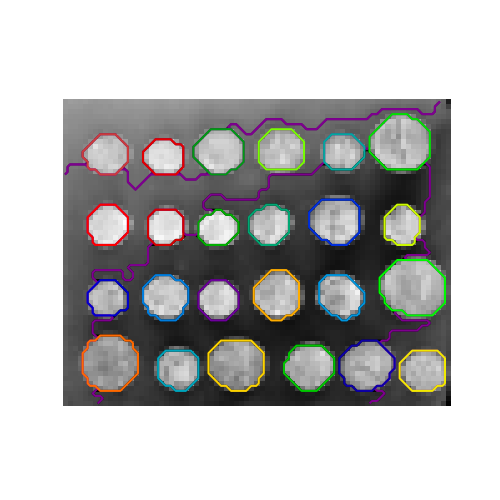#### 特征聚集(Feature agglomeration)

``````>>> digits = datasets.load_digits()
>>> images = digits.images
>>> X = np.reshape(images, (len(images), -1))
>>> connectivity = grid_to_graph(*images.shape)

>>> agglo = cluster.FeatureAgglomeration(connectivity=connectivity,
...                                      n_clusters=32)
>>> agglo.fit(X)
FeatureAgglomeration(connectivity=..., n_clusters=32)
>>> X_reduced = agglo.transform(X)

>>> X_approx = agglo.inverse_transform(X_reduced)
>>> images_approx = np.reshape(X_approx, images.shape)
``````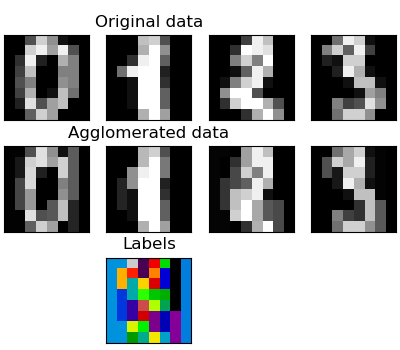`transform``inverse_transform`方法

## 分解: 从整个信号到其各个分量与负载

### 主成分分析: PCA``````>>> # 创建只有2个维度有用的信号
>>> x1 = np.random.normal(size=100)
>>> x2 = np.random.normal(size=100)
>>> x3 = x1 + x2
>>> X = np.c_[x1, x2, x3]

>>> from sklearn import decomposition
>>> pca = decomposition.PCA()
>>> pca.fit(X)
PCA()
>>> print(pca.explained_variance_)
[  2.18565811e+00   1.19346747e+00   8.43026679e-32]

>>> #如我们所见，只有前两个分量才有用
>>> pca.n_components = 2
>>> X_reduced = pca.fit_transform(X)
>>> X_reduced.shape
(100, 2)
``````

### 独立分量分析: ICA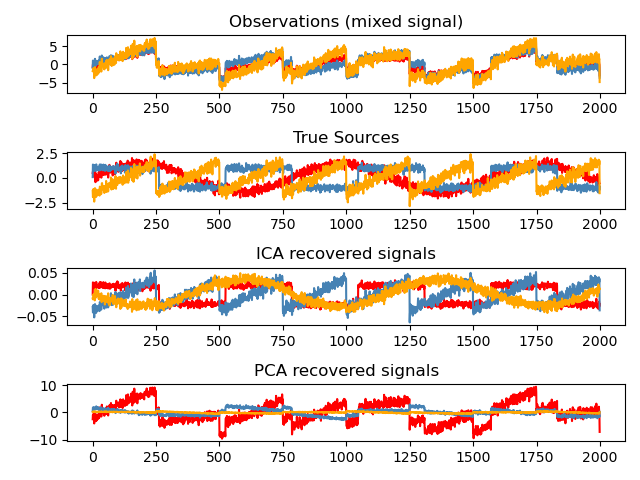``````>>> # 生成样本数据
>>> import numpy as np
>>> from scipy import signal
>>> time = np.linspace(0, 10, 2000)
>>> s1 = np.sin(2 * time)  # 信号1：正弦信号
>>> s2 = np.sign(np.sin(3 * time))  # 信号2：方波信号
>>> s3 = signal.sawtooth(2 * np.pi * time)  # 信号3：锯齿信号
>>> S = np.c_[s1, s2, s3]
>>> S += 0.2 * np.random.normal(size=S.shape)  # 添加噪音
>>> S /= S.std(axis=0)  # 标准化数据
>>> # 混合数据
>>> A = np.array([[1, 1, 1], [0.5, 2, 1], [1.5, 1, 2]])  # 混合矩阵
>>> X = np.dot(S, A.T)  # 生成观测数据

>>> # 计算 ICA
>>> ica = decomposition.FastICA()
>>> S_ = ica.fit_transform(X)  # 获取估计的来源
>>> A_ = ica.mixing_.T
>>> np.allclose(X,  np.dot(S_, A_) + ica.mean_)
True
``````